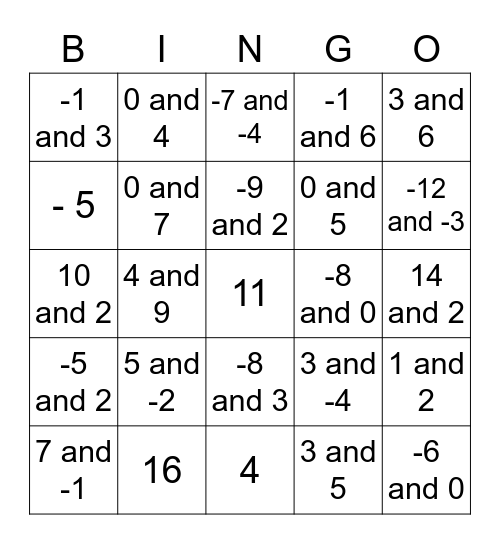This bingo card has 25 words: 5 and -2, 4, -9 and 2, 7 and -1, 3 and 6, -1 and 3, - 5, 0 and 4, -8 and 0, -8 and 3, 0 and 5, 10 and 2, 3 and -4, 1 and 2, 0 and 7, -6 and 0, 14 and 2, -7 and -4, -12 and -3, 4 and 9, 11, 16, -5 and 2, -1 and 6 and 3 and 5.

## Play Online